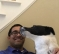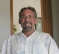## Hermitian matrixStarted by 6 years ago8 replieslatest reply 6 years ago579 views

I would like to clarify about Hermitian matrix. I am referring to the following URL to understand this:

http://mathworld.wolfram.com/HermitianMatrix.html

It is defined as A = Act where Act is conjugate transpose of A

A couple of examples are given A= [1, -i;i, 1]

I am converting A into Act by first finding conjugate of the elements and then transposing the matrix, as follows,

A_conjugate = [-1, i;-i, -1]

Transposing A_conjugate I get Act = [-1, -i; i, -1]

I don't find A = Act

Am I missing something?

Also, I would like to know some applications of Hermitian matrix.

[ - ]Hi,

You are negating the real part, you only flip the sign of the imaginary. Here is the MATLAB equivalent (https://www.mathworks.com/help/matlab/ref/ctranspo...) of what you are trying to do

>> A = [1, -i; i, 1]

A =

1.0000 + 0.0000i   0.0000 - 1.0000i

0.0000 + 1.0000i   1.0000 + 0.0000i

>> B = A'

B =

1.0000 + 0.0000i   0.0000 - 1.0000i

0.0000 + 1.0000i   1.0000 + 0.0000i

You have picked a conjugate symmetric matrix

If you pick a non symmetric matrix its clearer

>> A = [3 + 4i, 5 + 6i; -1 - 2i, -10 - 9i]

A =

3.0000 + 4.0000i   5.0000 + 6.0000i

-1.0000 - 2.0000i -10.0000 - 9.0000i

>> B = A'

B =

3.0000 - 4.0000i  -1.0000 + 2.0000i

5.0000 - 6.0000i -10.0000 + 9.0000i

>> C = ctranspose(A)

C =

3.0000 - 4.0000i  -1.0000 + 2.0000i

5.0000 - 6.0000i -10.0000 + 9.0000i

[ - ]thanks. I surely misunderstood matrix conjugate when element has only real form - a or a + 0*i

[ - ]Matrix conjugate is just the element-wise, plain old complex conjugate.  There's nothing special about the "matrix" part.

[ - ]thanks. Can anyone please let me know an application of Hermitian matrix?

[ - ]Nothing really specific, however in nearly all the applications in linear algebra where you'd take the transpose of a real-valued matrix, you take the Hermitian of a complex-valued matrix.

To the point where, in Scilab at least (and probably Matlab and Octave, but I couldn't be sure), A' takes the Hermetian of a complex matrix, not just its transpose.

[ - ]I believe we should start with the definition of an Hermitian Matrix.

This is a matrix whose conjugate transpose is equal (same as) the original matrix.

OK, so let's examine this:  in order for a transpose to have a chance to equal it's original matrix, the original must be square.  A little more thought requires that there be symmetry in the original.  That is, the i-th row, j-th column MUST be conjugate of the j-th row and i-th column, because after the transpose AND the conjugation, that is the only way to leave the same elements as the originas.  Lastly, since the transpose flips around the diagonal, that must be all reals only, otherwise the conjugation would change them.

Consequently, your matrix is NOT hermitian, since the diagonal is -1 i.  So, this is why the conjugate transpose is not the same.

Take a look at

[ 2    3-i 5+6i

3+i  5    1-i

5-6i  1+i  9 ]

Note the symmetry about the diagonal.  Note that thecdiagonal is all reals only.  And, note that it is square.

Take the conjugate transpose, and see what you get.

[ - ]thanks. Can you throw some light on the application of Hermitian matrix. I have seen this in the context of receive diversity in a wireless system where multiple receivers are present for receive diversity.

[ - ]Let me see if I can tackle this:

My first thought is, "are you taking a linear algebra class?"

If you're in the middle of a class, this won't make a lot of sense until nearly the end.

Linear Algebra has far more depth than most people attribute to it.  So, you need to understand what "spanning" means, what "subspaces" are, what "diagonalization" is, and means, well, and a few other things.

for the basics:

First, ALL matrices that are "Hermitian" have n independent "orthogonal" eigenvalues/eigenvectors.  This is important because it means that the set of eigenvectors "spans" the space.  And any subset, thereof, also spans that corresponding subspace.  Basically, that is to say, that ANY equation written using any of these vectors, will STAY within the same subspace.  I.E. linear modifications to any, will be still be "inside" the same subspace.  Very important.

Consequently, anything "done" to any of the receivers in your subspace remains in the subspace (or applies to them all).  In electronics, we rely upon linearity to allow us to apply "superposition".  Remembering that matrices allow us to solve/resolve multiple equations simultaneously, AND that with multiple receivers, though we are in the same space, we rely upon each subspace to apply to its respective receiver, we rely upon this very important property of the "Hermitian" to allow us to utilize "linear algebra" (or Hermitian matrices) to "simultaneously" solve the multiple equations involved with the "multiple" receivers.

I hope this helps.  I'm sorry if it this somewhat "outside" of the normal conversation of "dsprelated", this is really not a topic for this venue.  I'm still, "less than 'expert' with DSP", just happen to have some mathematical background.

Hope this helps,